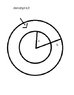# Eletric potential inside charged sphere with hole inside

• rmfw
In summary, the conversation is about finding the electric potential in all space for a charge density of ρ=k/r, k>0, located between two sphere surfaces of r=a and r=b, where b>a. The electric field equations for different regions are given, and the method for finding the electric potential is discussed. The key hint given is that V(r) for r<a is equal to V(r) for a<r<b.

## Homework Statement

Consider a charge density of ρ=k/r , k>0 , located between a sphere surface of r=a and another sphere surface of r=b, b>a.
I'm supposed to find the electric field on all space, which I did. Now I have to find the electric potential in all space, which I also did for r>b, but I'm having problems finding it for a<r<b and for r<a.

## Homework Equations

These are the electric field equations I came up with:
r<a : E(r)=0
r>b: E(r)=(k*(b2-a2))/(ε0*2*r2)
a<r<b: E(r)=(k*(1-a2/r2))/(2*ε0)

Electric potential for r>b: V(r)=(b2-a2)/(2ε0*r)

## The Attempt at a Solution

For finding the EP at r>b I just had to integrate E(r) for r>b with limits between r and ∞ which is equal to V(r)-V(∞) with V(∞)=0, but I can't come up with any solution for the other Epotentials, if someone could give me a hint I would appreciate.

Thanks!

#### Attachments

•sphere.jpg
11.1 KB · Views: 445
Based on your solution for r>b, what is V(b)?
Can you calculate the potential between a and b, if you know V(b)? The method is similar to the region r>b.
r<a is easy once you have the region a<r<b, as there is no field inside.

to find V(b) I can use the equation of the potential for r>b right?

Then to find V(r) for a<r<b:

V(r)=-∫rbE(r).dr + V(b)

and then repeat the process to find V(r) for r<a, where there is no field, which means V(r) for r<a = V(r) for a<r<b.

Correct?

Last edited:
rmfw said:
to find V(b) I can use the equation of the potential for r>b right?

Then to find V(r) for a<r<b:

V(r)=-∫rbE(r).dr + V(b)

and then repeat the process to find V(r) for r<a, where there is no field
Sure.

, which means V(r) for r<a = V(r) for a<r<b.

Correct?
I guess that is a typo here.

•1 person
I guess that is a typo here.

Ah yes! It should be V(r) = V(a) for r<a.

That was the hint I needed, thanks!